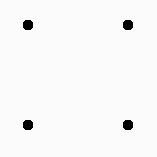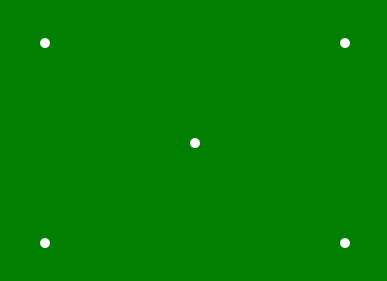Related Articles

# p5.js | point() Function

• Last Updated : 17 Jan, 2020

The point() function is an inbuilt function in p5.js which is used to draw the point in a given coordinate position.

Syntax:

`point( x, y, [z])`

Parameters: This function accept three parameters which are described below

• x: It is used to set the x-coordinate of point.
• y: It is used to set the y-coordinate of point.
• z: It is used to set the z-coordinate in WebGL mode.

Example 1:

 `function` `setup() {`` ` `    ``// Create Canvas of given size ``    ``createCanvas(400, 300);`` ` `}`` ` `function` `draw() {`` ` `    ``// Use point() function to draw point``    ``point(50, 50);``    ``point(150, 50);``    ``point(50, 150);``    ``point(150, 150);`` ` `    ``// Use strokeWeight() function to set``    ``// the weight of point``    ``strokeWeight(10);``}`

Output:Example 2:

 `function` `setup() { ``     ` `    ``// Create Canvas of given size ``    ``createCanvas(400, 300); ``} `` ` `function` `draw() { ``     ` `    ``// Set the background color``    ``background(``'green'``);``     ` `    ``// Set the stroke weight``    ``strokeWeight(10);`` ` `    ``// Set stroke color``    ``stroke(``'white'``);`` ` `    ``// Draw point``    ``point(50, 50);``   ` `    ``point(50, 250);``   ` `    ``point(350, 50);``   ` `    ``point(350, 250);``   ` `    ``point(200, 150);``   ` `} `

Output:Online editor: https://editor.p5js.org/
Environment Setup: https://www.geeksforgeeks.org/p5-js-soundfile-object-installation-and-methods/

Reference: https://p5js.org/reference/#/p5/point

Hey geek! The constant emerging technologies in the world of web development always keeps the excitement for this subject through the roof. But before you tackle the big projects, we suggest you start by learning the basics. Kickstart your web development journey by learning JS concepts with our JavaScript Course. Now at it’s lowest price ever!

My Personal Notes arrow_drop_up